# Remaining useful life (RUL) prediction of bearing by using regression model and principal component analysis (PCA) technique

## Apakrita Tayade1, Sangram Patil2, Vikas Phalle3, Faruk Kazi4, Satvasheel Powar5

1, 5Indian Institute of Technology, Mandi, India

2, 3, 4Veermata Jijabai Technological Institute, Mumbai, India

1Corresponding author

Vibroengineering PROCEDIA, Vol. 23, 2019, p. 30-36. https://doi.org/10.21595/vp.2019.20617
Received 1 March 2019; accepted 12 March 2019; published 25 April 2019

37th International Conference on Vibroengineering in Bratislava, Slovakia, April 25-26th, 2019

Views 3431
Abstract.

A wind turbine works under variable load and environmental conditions because of which failure rate has been on the rise. Failure of a gearbox, an integral part of producing wind energy, contributes to 80 % of the total downtime for the wind turbine. For ensuring better utilization of the wind turbines, Fault prognosis and condition monitoring of bearings are of utmost importance as it helps to reduce the downtime by early detection of faults which further increases the power output. In this paper, vibration signals produced and machine learning approach to determine the Remaining Useful Life (RUL) for a degraded bearing is studied. The methodology includes statistical feature extraction analysis with regression models. Further the feature selection is done using Principal Component Analysis (PCA) technique which produces training and testing sets which acts as an input parameter for regression models such as Support Vector Regressor (SVR) and Random Forest (RF). Weibull Hazard Rate Function is used for calculating the RUL of the bearing. Results This study shows the potential application of regression model as an effective tool for degradation performance prediction of bearing.

Keywords: remaining useful life, bearings, prognosis, random forest regression, support vector regression.

#### 1. Introduction

With the growing impact of climate change Renewable energy remains the only viable option to save the motherland. With India’s aim to achieve 175GW of installed capacity of renewable energy by 2020 wind energy provides one of the most potential sector. India has the 4th largest installed capacity of wind turbines in the world. We still lack to achieve to our full potentials due to unresolved failures like that of failure of a gearbox, an integral part of producing wind energy. Power output can be increased by early detection of faults by fault prognosis and condition monitoring. Prognostics forecast the performance of an element surveying the degree of deviation or degradation of a system from its typical operating conditions. The predicted time is known as the Remaining Useful Life (RUL), with accurate RUL reduction in the inspection as well as maintenance cost is observed, which further contributes in expanding the general proficiency of the plant. Predicting an approaching failure and estimating the RUL of a bearing is necessary for coming up with support and sidestepping sudden shutdowns of basic frameworks. This paper exhibits a hybrid method for prognosis of bearing which makes used of regression primarily based adaptive predictive models to gain proficiency with the advancing pattern tendency in a bearing’s health indicator. These models are then used to project forward in time and estimate the RUL of a bearing .

Prognostics is mainly distributed as: model-based prognostics and data-driven prognostics. Model-based prognostics attempts to incorporate physical modeling at material level while mathematical modeling at the system level with different system variables used into the estimation of RUL. Systems are complex, which arises the need for highly skilled labors, hence making it time-consuming and labor- intensive method. Whereas data-driven prognostics concentrates on the available system monitoring data. Here, failure prognosis includes prognostication of system degradation and time-to-failure supported on “state awareness” gathered from monitored information . The machine learning (ML) market is rising speedily in light of the Internet insurgency and deployment of ML improves the speed and exactness of capacities performed by the framework. A ton of research work is carried out on foreseeing the RUL of bearing utilizing a machine learning approach. However, the outcome appeared by the majority of this paper isn't remarkable. Developing interest for artificial intelligence and outstanding advancements in its improvement has encouraged plenty of researchers to use this approach in the prediction of bearing RUL. Traditional methods like Support Vector Machines (SVM) [3, 4] and Principal Component Analysis (PCA) [5, 6]. Nonetheless, SVM is sensitive to feature scaling and tend to overfit for big datasets. The prediction of feature scaled dataset has low accuracy due to limited available range in our case. Researchers have also used various forms of ANN for bearing RUL prediction [7, 8]. The ensemble of BP-ANN used by Zhang et al. has also been verified through experimental data . Ensembles are widely utilized in the domain of reliability in the past as they turn out to be vastly improved than alternate models. Stacking ensemble of ANN and Gradient Boosted Trees (GBT) work better than CNN, SVM, GBT, and MLP, as shown by Sandip et al. in his work on prognostics .

In this paper, Dataset utilized for the investigation is taken by IEEE PHM Data Challenge 2012 for FEMTO bearing informational collection . The methodology contains extraction of the statistical features in $X$ and $Y$ direction, features ranking is done utilizing a PCA approach which is furthermore used to make distinctive datasets. The appropriate scoring function is taken to figure the score of models using error between actual RULs and values predicted for test bearings.

#### 2.1. Support vector regression

The Support Vector Regression (SVR) utilizes similar principles because of the SVM for classification, with just a couple of minor contrasts. They depended on a process the loss function that overlooks errors, that settled within the certain distance of the true value. This function is known as ‘epsilon intensive’ loss function. The factors measure the expense of the errors on the input training points. The loss function is applied to correct errors which are more prominent than the threshold –$\epsilon$. Corresponding loss functions leads to the distributed illustration of the decision rule, giving significant algorithmic as well as illustrative preferences .

In SVR, the given input $X$ is initially mapped onto an $m$-dimensional feature space using some fixed (nonlinear) mapping, and then a linear model is built in this feature space. Utilizing mathematical code, the linear model $f\left(x,w\right)$ is given by:

(1)
$f\left(x,w\right)={\sum }_{j=1}^{m}{w}_{j}{g}_{j}\left(x\right)+b,$

where ${g}_{j}\left(x\right)$, $j=$ 1, …, $m$ defines a set of nonlinear transformations, where $b$ is the “bias” term. Often the data are assumed to have a mean value equal to zero, that the “$b$” is dropped.

#### 2.2. Random forest

The Random Forest (RF) is a standout amongst the best machine learning models for predictive analytics, creating its associate degree industrial workhorse for machine learning.

The RF modeling is a kind of additive model that forecasts by joining decisions from a sequence of base models. This model is written as per the equation:

(2)
$g\left(x\right)={f}_{0}\left(x\right)+{f}_{1}\left(x\right)+{f}_{2}\left(x\right)+\dots ,$

where the last model g is the summation of simple base models fi. Here, every base classifier is a straightforward decision tree . In RF, basic models are built independently utilizing an alternate subsample of the data. The RF model is extremely great at taking care of tabular dataset with numerical features or categorical features with less than many classes. In contrast to linear models, RF can catch non-linear activities amongst the features and the objective. One imperative argument is that tree-based models are not intended to deal with widely sparse features.

#### 3. Experimentation

The dataset used for the analysis is taken by IEEE PHM Data Challenge 2012 for FEMTO bearing data-set. Containing failure data of REB data obtained from a PRONOSTIA platform for 17 runs to failure. Collection of data is done by the test rig shown in the Fig. 1. Use of two accelerometers was done to gather the data. The useful life of the bearing is considered to end when the amplitude of the vibration signal reaches 20 g.

Fig. 1. Experimental set-up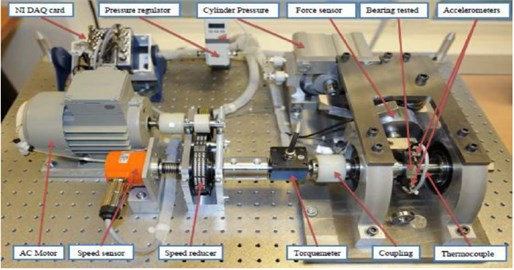Table 1. Bearing data-set 

 Data Condition 1 Condition 2 Condition 3 1800 rpm with 4000 N radial load 1650 rpm with 4200 N radial load 1500 rpm with 5000 N radial load Training data (6) 1_1 2_1 3_1 1_2 2_2 3_2 Testing data (11) 1_3 2_3 3_3 1_4 2_4 1_5 2_5 1_6 2_6 1_7 2_7

#### 3.1. Feature extraction

Raw data is pre-processed, and different time-domain features were calculated to smoothen the noisy, inconsistent and long data set.

#### 4. Methodology

The proposed methodology in this paper is shown in Fig. 2.

STEP 1: Input data is acquired by IEEE PHM Data challenge 2012 - FEMTO bearing data set.

STEP 2: Target function – Assumption: Last cycle = Failure cycle:

(3)

STEP3: Extraction of statistical features and doing feature ranking Fig. 3.by PCA method. Ranking of RPM and load are considered as immaterial.

STEP 4: Datasets were created using their rank which were further used to create data frames for regression analysis.

STEP 5: Similar datasets were created for the testing data and RUL is predicted for each test bearing. Error and score calculations are done for the predicted RUL.

Table 2. Statistical features

 Sr. No. Mathematical formulations 1 Standard deviation $\left({\sigma }_{a}\right)=\sqrt{\frac{\sum _{n=1}^{N}\left(a\left(n\right){-{\mu }_{a}\right)}^{2}}{N-1}}$ 2 Mean $\left({a}_{mean}\right)=\frac{\sum _{n=1}^{N}a\left(n\right)}{N}$ 3 Variance $\left({\sigma }_{a}^{2}\right)=\frac{\sum _{n=1}^{N}\left(a\left(n\right){-{\mu }_{a}\right)}^{2}}{N-1}$ 4 Skewness $\left({SK}_{a}\right)=\frac{\sum _{n=1}^{N}\left(a\left(n\right){-{\mu }_{a}\right)}^{3}}{\left(N-1\right){\sigma }_{a}^{3}}$ 5 Root mean square error $\left({a}_{rms}\right)=\sqrt{\frac{\sum _{n=1}^{N}\left(a\left(n\right){\right)}^{2}}{N-1}}$ 6 Kurtosis ${\left(K}_{a}\right)=\frac{\sum _{n=1}^{N}\left(a\left(n\right){-{\mu }_{a}\right)}^{4}}{\left(N-1\right){\sigma }_{a}^{4}}$

Fig. 2. Methodology used for prediction of RUL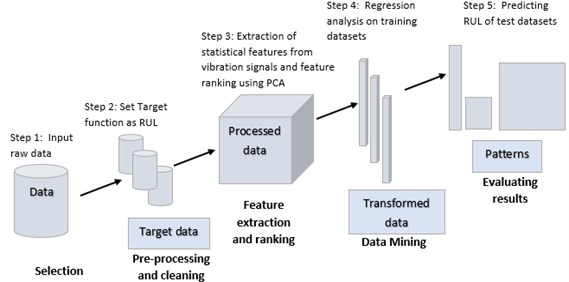#### 4.1. Feature ranking and feature set formation

Principal component analysis (PCA) is used to recognize the contribution of features that are most adding to the principal components. It uses a variance ratio for ranking the given features. Variance is calculated using:

(4)
$\sigma =\sum j\lambda j.$

So, variance ratio ($rj$) for the given component $j$ is calculated the following formula:

(5)

Using ranked features, 12 feature sets were made so that the first feature set contains the first top-ranked feature, at that point the second feature set contains the top two ranked features and so on. It enables to test the importance of features and the overall accuracy of the system.

Fig. 3. Feature ranking using PCA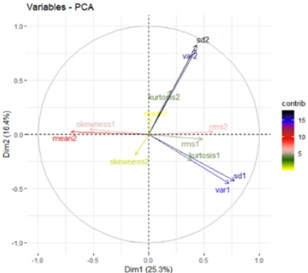#### 5. Result

RUL is calculated for the proposed model and results obtained are discussed below. Error in the predicted and actual RUL is calculate using following formula:

(6)

The scoring function used is as follows:

(7)

The overall score is calculated using:

(8)

Fig. 4. Trend of scores of feature datasets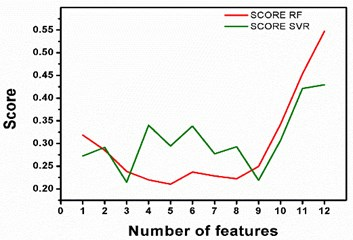Fig. 5. Comparison between regression models for 12 features dataset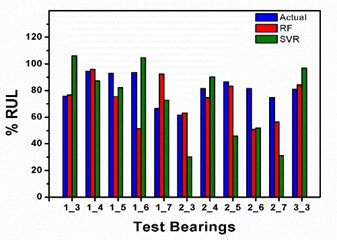In the present study SVR and RF models are employed to predict RUL of bearing. firstly, features are extracted from IEEE PHM Data Challenge 2012 bearing dataset and ranked using PCA, which are further used to form training and testing input feature sets. Fig. 4. depicts the graph of calculated scores using SVR and RF as per mathematical expression given in data sheet. For RF regression model, the score is indicating lessening pattern till 8 features dataset however it rockets up with the expansion in the features and finally both the models achieve highest score with 12th feature set and score with RF outperforms SVR. Fig. 5.

Displays comparison between actual RUL and RUL computed using the regression models of IEEE FAMTO data set. Fig. 5. demonstrates the relative investigation between the 2 models using 12 features datasets with the actual value anticipated. The score of the SVR model is 0.429582 which is most reduced while the RF model is indicating most astounding score estimation of 0.547452. Table 3 contains the values of bearing’s actual RUL and predicted RUL to calculate score. The highest score of 0.547452 is achieved with 12th feature set and RF.

Table 3. Predicted life and score calculation for a dataset with 12 features

 Test bearings Actual life RF SVR Predicted life Percentage error Predicted life Percentage error 1_3 75.86 76.6527 –1.0449 107.687 –41.9552 1_4 94.48 95.7513 –1.3455 87.6651 7.21298 1_5 93 75.3283 19.0017 85.0334 8.56620 1_6 93.65 51.5245 44.9818 106.247 –13.4515 1_7 66.47 92.3060 –38.8687 72.9294 –9.7179 2_3 61.48 63.1857 –2.7744 63.1718 –2.7518 2_4 81.46 74.6707 8.33449 88.9595 –9.2064 2_5 86.62 83.4768 3.62872 83.6839 3.38957 2_6 81.57 50.8047 37.7163 53.3920 34.5444 2_7 74.67 56.3326 24.5578 30.8943 58.6255 3_3 81.06 84.1921 –3.8639 85.0971 –4.9803 Score 0.547452 0.429582

#### 6. Conclusions

Dataset utilized for the investigation is taken by IEEE PHM Data Challenge 2012 for FEMTO bearing informational collection. The methodology contains extraction of the statistical features in $X$ and $Y$ direction, features are ranked using a PCA approach which is additionally used to make distinctive datasets utilizing feature ranking. The outcome demonstrates RF regression is more exact than SVR models. The best score 0.5474 is accomplished utilizing RF regression mode with 12 features dataset. The result indicates the potential use of group regression procedure for a forecast of RUL.

#### Acknowledgements

Authors would like to acknowledge Larsen and Toubro Infotech Ltd. funding under CSR-1step initiative.

1. Ahmad W., Ali Khan S., Kim J. M. A hybrid prognostics technique for rolling element bearings using adaptive predictive models. IEEE Transactions on Industrial Electronics, Vol. 65, Issue 2, 2017, p. 1577-1584. [Publisher]
2. Sankavaram C., et al. Model-based and data-driven prognosis of automotive and electronic systems. IEEE International Conference on Automation Science and Engineering, 2009. [Publisher]
3. Soualhi A., Medjaher K., Zerhouni N. Bearing health monitoring based on Hilbert-Huang transform, support vector machine, and regression. IEEE Transactions on Instrumentation and Measurement, Vol. 64, Issue 1, 2015, p. 52-62. [Publisher]
4. Sloukia F., Aroussi M. E., Medromi H., Wahbi M. Bearings prognostic using mixture of Gaussians hidden Markov model and support vector machine. ACS International Conference on Computer Systems and Applications, 2013. [Publisher]
5. Wang F., Chen X., Liu C., Yan D., Han Q., Li H. Reliability assessment of rolling bearing based on principal component analysis and Weibull proportional hazard model. IEEE International Instrumentation and Measurement Technology Conference, 2017. [Publisher]
6. Zhao M., Tang B., Tan Q. Bearing remaining useful life estimation based on time-frequency representation and supervised dimensionality reduction. Measurement, Vol. 86, 2016, p. 41-55. [Publisher]
7. Guo L., Guo H., Huang H., He X., Li S. Multifeatures fusion and nonlinear dimension reduction for intelligent bearing condition monitoring. Shock and Vibration, Vol. 2016, 2016, p. 4632562. [Publisher]
8. Zurita D., Carino J. A., Delgado M., Ortega J. A. Distributed neuro-fuzzy feature forecasting approach for condition monitoring. IEEE Conference on Emerging Technology and Factory Automation, Barcelona, 2014. [Publisher]
9. Zhanga Bingsen, Zhang Lijun, Xub Jinwu Remaining useful life prediction for rolling element bearing based on ensemble learning. Chemical Engineering Transactions, Vol. 33, 2013, p. 157-162. [Search CrossRef]
10. Singh Sandip Kumar, Kumar Sandeep, Dwivedi J. P. A novel soft computing method for engine RUL prediction. Multimedia Tools and Application, Vol. 78, Issue 4, 2019, p. 4065-4087. [Publisher]
11. IEEE PHM 2012 Prognostic Challenge. Outline, Experiments, Scoring of Results, Winners, 2012. [Search CrossRef]
12. Prosvirin A., Islam M. M. M., Kim C., Kim J. M. Fault prediction of rolling element bearings using one class least squares SVM. The Engineering and Arts Society in Korea, EASKO, 2017. [Search CrossRef]
13. Aggarwal C. C. Data mining: The Textbook. Springer, Berlin, Germany, 2015. [Publisher]

#### Cited By

 Journal of Physics: Conference Series Wanwan Zhang, Jørn Vatn, Adil Rasheed 2022 Russian Journal of Nondestructive Testing M. Riahi, A. Maghsoudi 2022 Journal of Computing and Information Science in Engineering Bin He, Long Liu, Dong Zhang 2021 International Journal of System Assurance Engineering and Management Nikhil M. Thoppil, V. Vasu, C. S. P. Rao 2021 IEEE Sensors Journal Danyang Han, Jinsong Yu, Mengtong Gong, Yue Song, Limei Tian 2021 Computational Intelligence and Neuroscience Honglin Luo, Lin Bo, Xiaofeng Liu, Hong Zhang, Heng Liu 2021 Eksploatacja i Niezawodność – Maintenance and Reliability Gao Zhiyong, Li Jiwu, Wang Rongxi 2021 Frontiers in Artificial Intelligence Luca Biggio, Iason Kastanis 2020